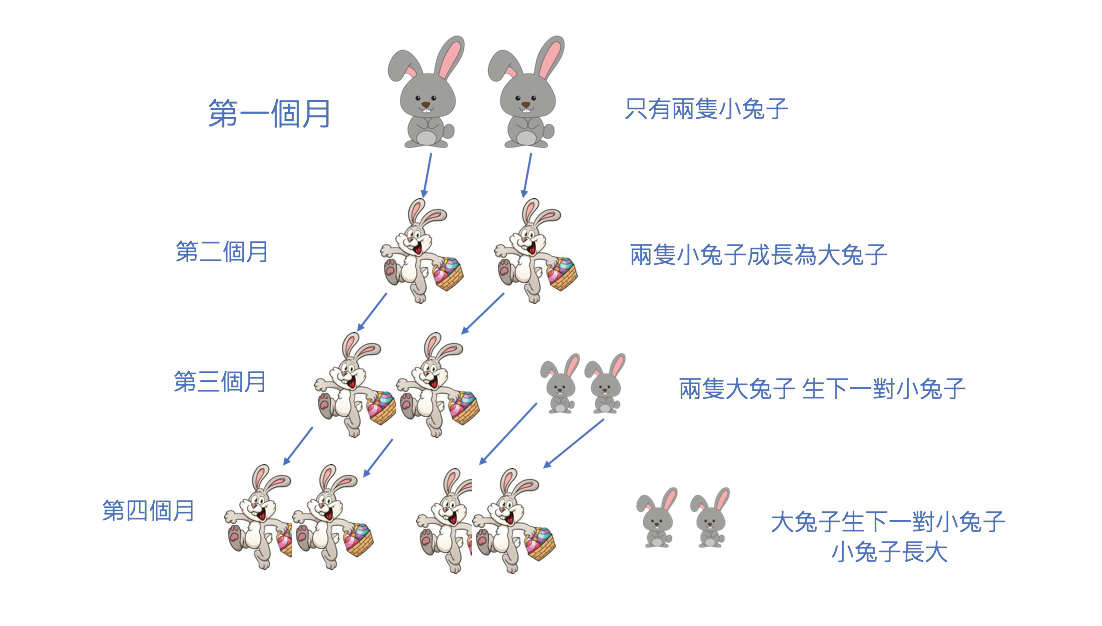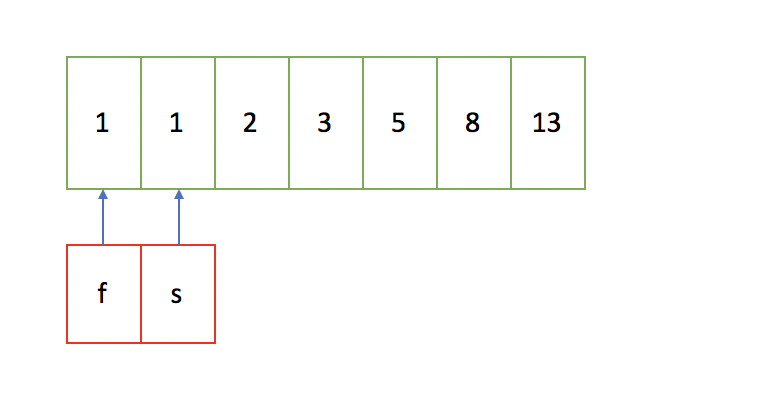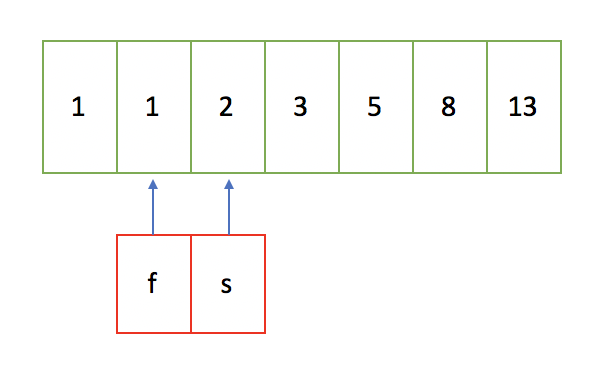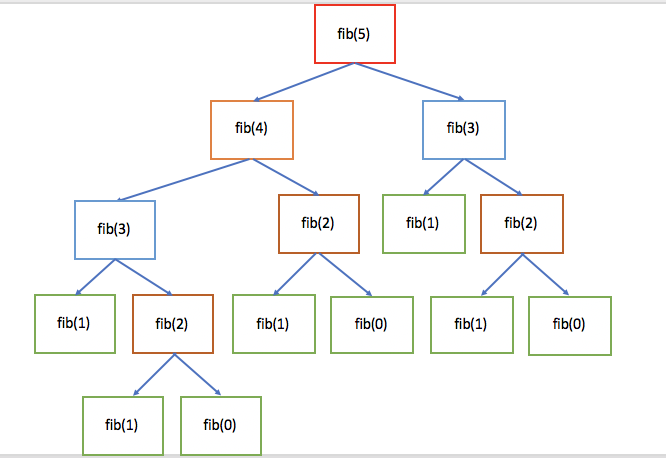# Day 02 七天學會基本演算法（二）淺談演算法複雜度與費波那契數列## 如何評判一個演算法的好壞？

（一）迭代算法

``````int sum = 0; // 計算一次
for(int i = 1; i <= n ;i++){
sum += i; // 共計算了n次
}
``````

（二）數學公式

``````(1 + n) * n / 2; // 計算一次
``````

### BigO

• 常忽略常數、係數，因為當n大到一定的程度時，我們可以不用管它
• 9 表示為 O(1)
• 2n+3 表示為O(n)
• n^2 + 2n + 6 表示為O(n^2) 因為當n非常大時，2n是影響不大的

(1)

``````public static void test1(int n) {
// 1次計算
if (n > 10) {
System.out.println("n > 10");
} else if (n > 5) { // 2
System.out.println("n > 5");
} else {
System.out.println("n <= 5");
}
// 4次計算
for (int i = 0; i < 4; i++) {
System.out.println("test");
}

// 共5次計算 => Ｏ(1)

}
``````

(2)

``````public static void test2(int n) {
for (int i = 0; i < n; i++) { // n次
for (int j = 0; j < 15; j++) { // 常數次
System.out.println("test");
}
}
// O(n*常數) => O(n)
}
``````

(3) 指數

``````public static void test3(int n) {
for (int i = 1; i < n; i = i * 2) {
// i = 1, 2,4,8,16 = 2^k
//  2^k > n
// k > log2(n)
// 因此時間複雜度為O(logn);
System.out.println("test");
}
}
``````

## 費波那契級數（Fibonacci)

• 第一個月有一對兔子誕生
• 過了一個月後，他們會變成成年的兔子，可以進行生育
• 之後的每月，每一對都可生育一對兔子
• 兔子不會死亡``````fibonacci: 1, 1, 2, 3, 5, 8....
``````

``````fib(0) = 1 // 第一個月只有一對兔子
fib(1) = 1 // 第二個月也只有一對兔子
fib(2) = 2
fib(3) = 3 = fib(1) + fib(2)
fib(4) = 5 = fib(3) + fib(2)
.
.
.
fib(n) = fib(n-1) + fib(n-2)
``````

（ㄧ）使用遞迴的方式

``````private int fib1(int n){
if(n <= 1){
return n;
}
return fib1(n-1)+ fib(n-2);
}
``````

（二）使用迭代的方式``````private int fib2(int n){
if(n <= 1){
return n;
}
int first = 1;
int second = 1;
int sum = 0;
for(int i = 0; i < n-1; i++){
sum = first + second; // sum為前兩個數字相加
first = second; // 運算完sum之後，first移到second的位置
second = sum; // second移到first的位置
}
return sum;
}
``````

### 演算法的比較